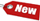Browse Accounting Lessons HereAccounting Terms & DefinitionsAccounting for Merchandising ActivitiesDebits and Credits (Double Entry Accounting)Business Valuation FormulasTime Value of Money & Present/Future ValuesComplex Debt & Equity InstrumentsCommon Stock & Shareholder's EquityAccounting & Finance RatiosValuing Common StockCorporate Income TaxesLower of Cost or Market (LCM) & Inventory ValuationChart of Accounts & BookkeepingBonds Payable & Long Term LiabilitiesCapital AssetsGAAP, Accrual & Cash Accounting, Information Commodity, Internal Controls & MaterialityWhat category of browser are you on this website? Accounting student (homework help) Finance professor (university research) Accounting manager (at work) Other Explore Careers in Accounting and FinanceVisit our section on Careers in Accounting & Finance to explore vast opportunities in this industry.

Chapter 4.17® - Accounting for Perpetuities & Using the Annuity Present Value Factor Formula

From the chapter on present value of steady annuity cash flows, we know that we can find the present value of future steady annuity payments. However, what happens when the level of future cash flows is not for a definite period of time, but forever? Such an asset is known as perpetuity. Thus simply defined, perpetuity is an annuity in which the cash flows continue forever. Some perpetuity is also known as consols.

Since perpetuity has infinite number of cash flows, we can’t find its value by discounting each flow to its present value state. However, valuing perpetuity is very easy; consider this example. Say perpetuity costs \$2000 and offers a 15% rate of return with payments at the end of the year. The cash flow each year must be:

 Cash flow = \$2,000 x 15% = \$300

To define this in terms of an equation, the present value of perpetuity (PV = \$2,000) multiplied by the rate (r = 15%) = \$300. The formula then is:

 Perpetuity present value x Rate = Cash flow PV x r = C

Therefore, given a cash flow and a rate of return, we can easily calculate the present value for the perpetuity:

 PV for Perpetuity = C / r = C x (1 / r)

For instance, an investment offers a perpetual cash flow of \$1000 every year. The return you would expect from such an investment is 10% a year. What is the value of this investment? The value of this perpetuity will be:

 Perpetuity PV = C x (1 / r) Perpetuity PV = \$1,000 x (1 / 0.10) Perpetuity PV = \$1,000 x 10 Perpetuity PV = \$10,000

Consider another example where an investment offers a perpetual cash flow of \$4,000 per year and the return rate you should expect is 18% a year. What is the present value of this perpetuity?

 Perpetuity PV = C x (1 / r) Perpetuity PV = \$4,000 x (1 / 0.18) Perpetuity PV = \$4,000 x 5.56 Perpetuity PV = \$22,240

Another way of looking at perpetuity’s value and why it is easy to determine is by taking into account the expression for an annuity present value factor:

 Annuity present value factor = (1 – Present value factor) / r Annuity present value factor = (1 / r) x (1 – Present value factor) Annuity present value factor = (1 / r) x (1 – (\$1/ (1 + r)t)

Let’s revisit our previous example; an investment offers a perpetual cash flow of \$1000 every year for 100 years. The return you would expect from such an investment is 10% a year. What is the value of this investment? The value of this perpetuity will be:

 Annuity present value factor = (1 / r) x (1 – Present value factor) Annuity present value factor = (1 / r) x (1 – (\$1/ (1 + r)t) Annuity present value factor = (1 / 0.10) / (1 – (\$1 / (1 + 0.10100) Annuity present value factor = (1 / 0.10) / (1 – (\$1 / (1.1100) Annuity present value factor = (1 / 0.10) / (1 – 0.0000726) Annuity present value factor = 10 / 0.9999274 Annuity present value factor = 10.0007 Perpetuity PV = \$1,000 x 10.000 Perpetuity PV = \$10,000

Suppose we did this calculation using our BAII Plus financial calculator. The table would look like:

 N = 100 I/Y = 10% PV = ? PMT = \$1,000 FV = \$0 2ND I/Y P/Y = 1 C/Y = 1 CPT & PV = \$9,999.27

This amount of \$9,999.27 is pretty close to the \$10,000 we derived using our annuity present value factor formula.